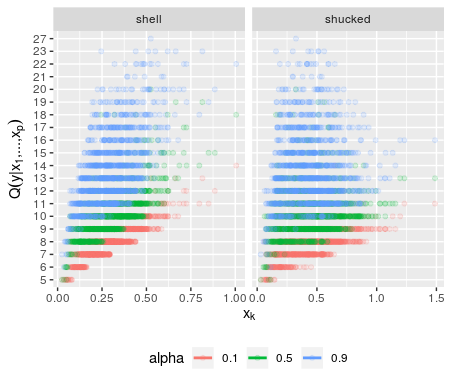# Example usage of the vinereg package

#### September 2017

This file contains the source code of an exemplary application of the D-vine copula based quantile regression approach implemented in the R-package vinereg and presented in Kraus and Czado (2017): D-vine copula based quantile regression, Computational Statistics and Data Analysis, 110, 1-18. Please, feel free to address questions to daniel.kraus@tum.de.

library(vinereg)
library(ggplot2)
library(dplyr)
library(tidyr)

# Data analysis

set.seed(5)

We consider the data set abalone from the UCI Machine Learning Repository (https://archive.ics.uci.edu/ml/datasets/abalone) and focus on the female sub-population. In a first application we only consider continuous variables and fit models to predict the quantiles of weight (whole) given the predictors length, diameter, and height.

data(abalone, package = "PivotalR")
abalone_f <- abalone %>%
dplyr::filter(sex == "F") %>%        # select female abalones
dplyr::select(-id, -sex) %>%         # remove id and sex variables
dplyr::filter(height < max(height))  # remove height outlier
pairs(abalone_f, pch = ".")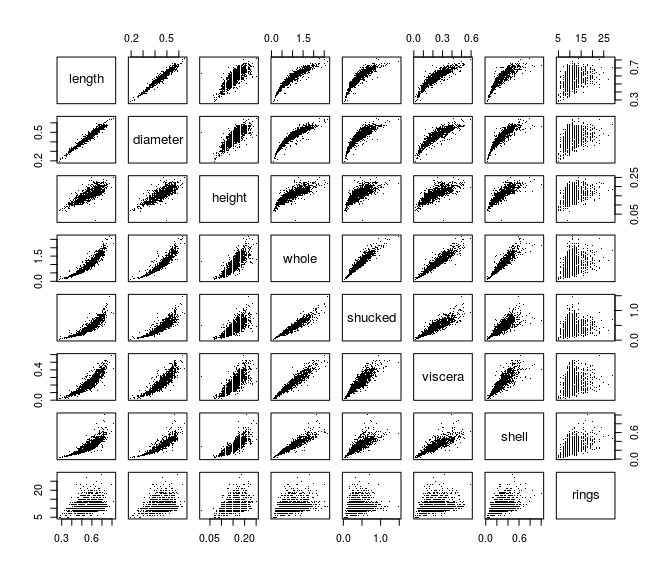# D-vine regression models

## Parametric D-vine quantile regression

We consider the female subset and fit a parametric regression D-vine for the response weight given the covariates len, diameter and height (ignoring the discreteness of some of the variables). The D-vine based model is selected sequentially by maximizing the conditional log-likelihood of the response given the covariates. Covariates are only added if they increase the (possibly AIC- or BIC-corrected) conditional log-likelihood.

We use the function vinereg() to fit a regression D-vine for predicting the response weight given the covariates length, diameter, and height. The argument family_set determines how the pair-copulas are estimated. We will only use one-parameter families and the t copula here. The selcrit argument specifies the penalty type for the conditional log-likelihood criterion for variable selection.

(fit_vine_par <- vinereg(
whole ~ length + diameter + height,
data = abalone_f,
selcrit = "aic"
))
## D-vine regression model: whole | length, height, diameter
## nobs = 1306, edf = 20.73, cll = 1092.08, caic = -2142.69, cbic = -2035.41

The result has a field order that shows the selected covariates and their ranking order in the D-vine.

fit_vine_par$order ##  "length" "height" "diameter" The field vine contains the fitted D-vine, where the first variable corresponds to the response. The object is of class "vinecop_dist" so we can use rvineocpulib’s functionality to summarize the model summary(fit_vine_par$vine)
## # A data.frame: 6 x 9
##   tree edge conditioned conditioning family rotation  parameters df  tau
## 1    1    1        1, 2              gumbel      180         5.1  1 0.80
## 2    1    2        2, 4              gumbel      180         2.3  1 0.57
## 3    1    3        4, 3              gumbel      180         2.4  1 0.58
## 4    2    1        1, 4            2      t        0 0.45, 15.23  2 0.30
## 5    2    2        2, 3            4      t        0  0.91, 4.59  2 0.74
## 6    3    1        1, 3         4, 2      t        0  0.32, 8.26  2 0.20

We can also plot the contours of the fitted pair-copulas.

contour(fit_vine_par$vine)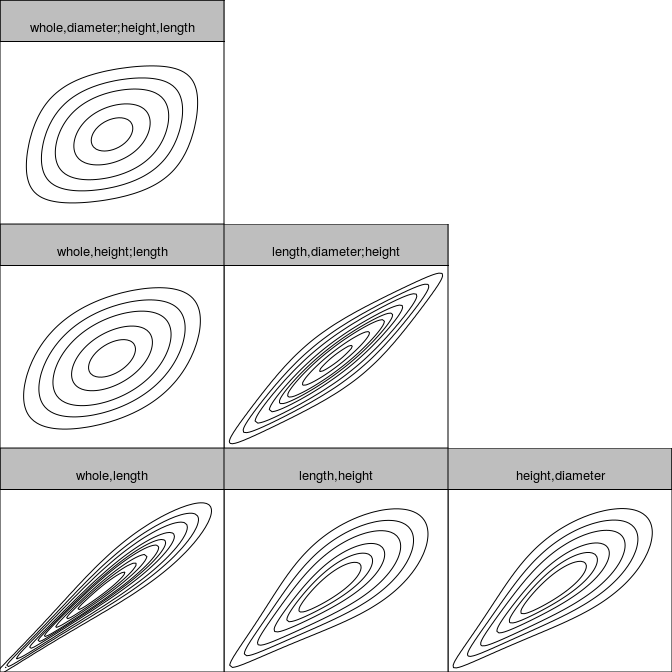## Estimation of corresponding conditional quantiles In order to visualize the predicted influence of the covariates, we plot the estimated quantiles arising from the D-vine model at levels 0.1, 0.5 and 0.9 against each of the covariates. # quantile levels alpha_vec <- c(0.1, 0.5, 0.9)  We call the fitted() function on fit_vine_par to extract the fitted values for multiple quantile levels. This is equivalent to predicting the quantile at the training data. The latter function is more useful for out-of-sample predictions. pred_vine_par <- fitted(fit_vine_par, alpha = alpha_vec) # equivalent to: # predict(fit_vine_par, newdata = abalone.f, alpha = alpha_vec) head(pred_vine_par) ## 0.1 0.5 0.9 ## 1 0.6566616 0.7600738 0.8732819 ## 2 0.6826392 0.7860813 0.9030168 ## 3 0.6619671 0.7761635 0.8924578 ## 4 0.7623786 0.8732481 0.9929738 ## 5 0.5812824 0.6890220 0.8231798 ## 6 0.6600508 0.7663987 0.8852566 To examine the effect of the individual variables, we will plot the predicted quantiles against each of the variables. To visualize the relationship more clearly, we add a smoothed line for each quantile level. This gives an estimate of the expected effect of a variable (taking expectation with respect to all other variables). plot_effects(fit_vine_par) ## geom_smooth() using method = 'gam' and formula 'y ~ s(x, bs = "cs")'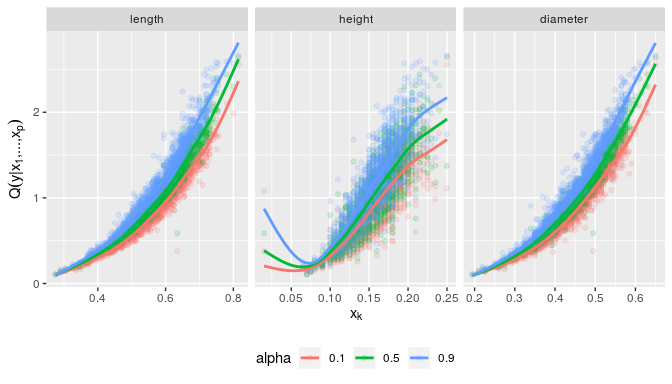The fitted quantile curves suggest a non-linear effect of all three variables. ## Comparison to the benchmark model: linear quantile regression This can be compared to linear quantile regression: pred_lqr <- pred_vine_par for (a in seq_along(alpha_vec)) { my.rq <- quantreg::rq( whole ~ length + diameter + height, tau = alpha_vec[a], data = abalone_f ) pred_lqr[, a] <- quantreg::predict.rq(my.rq) } plot_marginal_effects <- function(covs, preds) { cbind(covs, preds) %>% tidyr::gather(alpha, prediction, -seq_len(NCOL(covs))) %>% dplyr::mutate(prediction = as.numeric(prediction)) %>% tidyr::gather(variable, value, -(alpha:prediction)) %>% ggplot(aes(value, prediction, color = alpha)) + geom_point(alpha = 0.15) + geom_smooth(method = "gam", formula = y ~ s(x, bs = "cs"), se = FALSE) + facet_wrap(~ variable, scale = "free_x") + ylab(quote(q(y* "|" * x * ",...," * x[p]))) + xlab(quote(x[k])) + theme(legend.position = "bottom") } plot_marginal_effects(abalone_f[, 1:3], pred_lqr)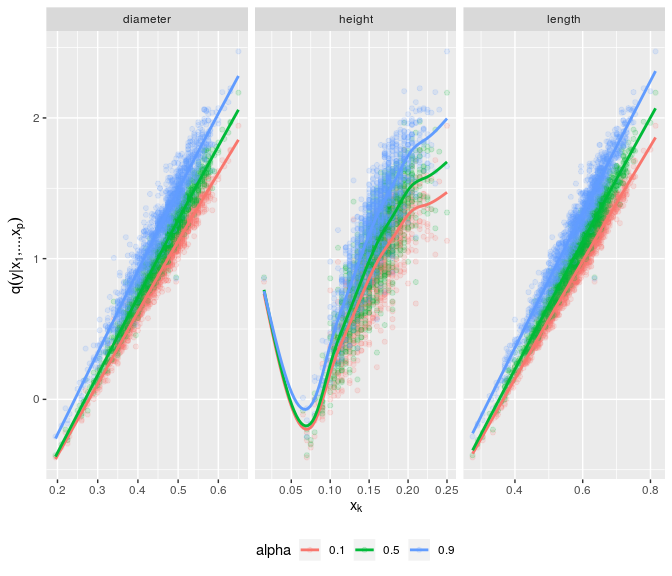## Nonparametric D-vine quantile regression We also want to check whether these results change, when we estimate the pair-copulas nonparametrically. (fit_vine_np <- vinereg( whole ~ length + diameter + height, data = abalone_f, family_set = "nonpar", selcrit = "aic" )) ## D-vine regression model: whole | length, height ## nobs = 1306, edf = 144.38, cll = 1136.86, caic = -1984.97, cbic = -1237.86 contour(fit_vine_np$vine)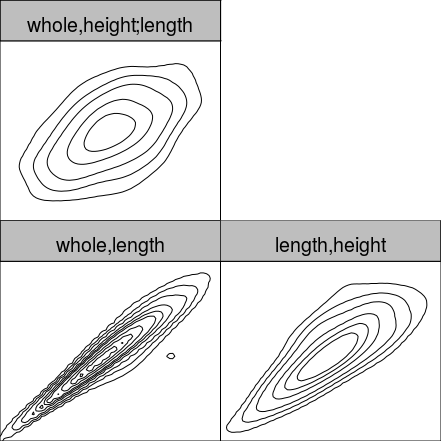Now only the length and height variables are selected as predictors. Let’s have a look at the marginal effects.

plot_effects(fit_vine_np, var = c("diameter", "height", "length"))
## geom_smooth() using method = 'gam' and formula 'y ~ s(x, bs = "cs")'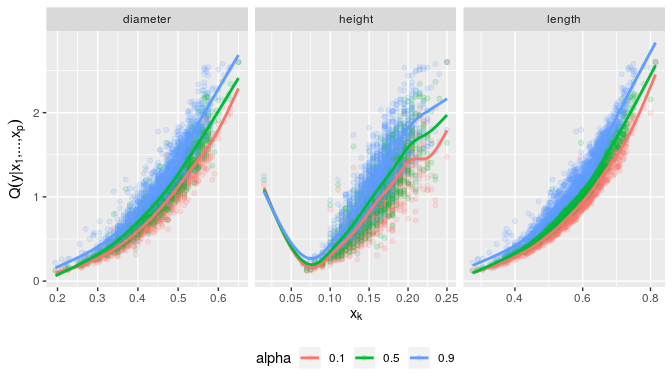The effects look similar to the parametric one, but slightly more wiggly. Note that even the diameter was not selected as a covariate, it’s marginal effect is captured by the model. It just does not provide additional information when height and length are already accounted for.

## Discrete D-vine quantile regression

To deal with discrete variables, we use the theory which is developed in Nagler (2017) and applied to D-vine quantile regression in Schallhorn et al. (2017). For the estimation the discrete variable(s) are transformed to continuous ones by jittering.

We let vinereg() know that a variable is discrete by declaring it ordered.

abalone_f$rings <- as.ordered(abalone_f$rings)
(fit_disc <- vinereg(
rings ~ .,
data = abalone_f,
family_set = "nonpar",
selcrit = "aic"
))
## D-vine regression model: rings | shell, shucked
## nobs = 1306, edf = 114.32, cll = -2774.44, caic = 5777.53, cbic = 6369.12
plot_effects(fit_disc)
## geom_smooth() using method = 'loess' and formula 'y ~ x'